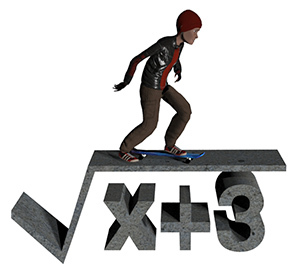• Browse All Lessons

Assign Lesson

Help Teaching subscribers can assign lessons to their students to review online!

 Tweet

Introduction:Have you ever become stuck while trying to solve geometry questions which involve exponents and/or radicals while trying to solve geometry problems such as the surface area of a sphere? It can be difficult to solve problems involving radicals and rational expressions unless you learn a few tricks. This lesson will help you to become confident solving these types of questions.

One of the main things to watch out for is that an equation is never fully simplified as long as their is a radical in the denominator. This is at least partly because it makes the equation easier to work with. For instance it is much easier to closely approximate $sqrt2/2$ doing mental math than with its equivalent fraction $1/sqrt2$.

Directions for This Lesson: